# 14.4 Rl circuits  (Page 3/4)

 Page 3 / 4

Check Your Understanding Verify that RC and L/R have the dimensions of time.

Check Your Understanding (a) If the current in the circuit of in [link] (b) increases to $90\text{%}$ of its final value after 5.0 s, what is the inductive time constant? (b) If $R=20\phantom{\rule{0.2em}{0ex}}\text{Ω}$ , what is the value of the self-inductance? (c) If the $20\text{-}\text{Ω}$ resistor is replaced with a $100\text{-}\text{Ω}$ resister, what is the time taken for the current to reach $90\text{%}$ of its final value?

a. 2.2 s; b. 43 H; c. 1.0 s

Check Your Understanding For the circuit of in [link] (b), show that when steady state is reached, the difference in the total energies produced by the battery and dissipated in the resistor is equal to the energy stored in the magnetic field of the coil.

## Summary

• When a series connection of a resistor and an inductor—an RL circuit—is connected to a voltage source, the time variation of the current is
$I\left(t\right)=\frac{\text{ε}}{R}\left(1-{e}^{\text{−}Rt\text{/}L}\right)=\frac{\text{ε}}{R}\left(1-{e}^{\text{−}t\text{/}{\tau }_{L}}\right)$ (turning on),
where the initial current is ${I}_{0}=\epsilon \text{/}R.$
• The characteristic time constant $\tau$ is ${\tau }_{L}=L\text{/}R,$ where L is the inductance and R is the resistance.
• In the first time constant $\tau ,$ the current rises from zero to $0.632{I}_{0},$ and to 0.632 of the remainder in every subsequent time interval $\tau .$
• When the inductor is shorted through a resistor, current decreases as
$I\left(t\right)=\frac{\epsilon }{R}{e}^{\text{−}t\text{/}{\tau }_{L}}$ (turning off).
Current falls to $0.368{I}_{0}$ in the first time interval $\tau$ , and to 0.368 of the remainder toward zero in each subsequent time $\tau .$

## Conceptual questions

Use Lenz’s law to explain why the initial current in the RL circuit of [link] (b) is zero.

As current flows through the inductor, there is a back current by Lenz’s law that is created to keep the net current at zero amps, the initial current.

When the current in the RL circuit of [link] (b) reaches its final value $\text{ε}\text{/}R,$ what is the voltage across the inductor? Across the resistor?

Does the time required for the current in an RL circuit to reach any fraction of its steady-state value depend on the emf of the battery?

no

An inductor is connected across the terminals of a battery. Does the current that eventually flows through the inductor depend on the internal resistance of the battery? Does the time required for the current to reach its final value depend on this resistance?

At what time is the voltage across the inductor of the RL circuit of [link] (b) a maximum?

At $t=0$ , or when the switch is first thrown.

In the simple RL circuit of [link] (b), can the emf induced across the inductor ever be greater than the emf of the battery used to produce the current?

If the emf of the battery of [link] (b) is reduced by a factor of 2, by how much does the steady-state energy stored in the magnetic field of the inductor change?

1/4

A steady current flows through a circuit with a large inductive time constant. When a switch in the circuit is opened, a large spark occurs across the terminals of the switch. Explain.

Describe how the currents through ${R}_{1}\phantom{\rule{0.2em}{0ex}}\text{and}\phantom{\rule{0.2em}{0ex}}{R}_{2}$ shown below vary with time after switch S is closed.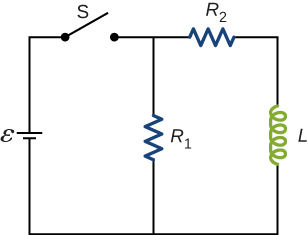Initially, ${I}_{R1}=\frac{\epsilon }{{R}_{1}}$ and ${I}_{R2}=0$ , and after a long time has passed, ${I}_{R1}=\frac{\epsilon }{{R}_{1}}$ and ${I}_{R2}=\frac{\epsilon }{{R}_{2}}$ .

Discuss possible practical applications of RL circuits.

## Problems

In [link] , $\epsilon =12\phantom{\rule{0.2em}{0ex}}\text{V}$ , $L=20\phantom{\rule{0.2em}{0ex}}\text{mH}$ , and $R=5.0\phantom{\rule{0.2em}{0ex}}\text{Ω}$ . Determine (a) the time constant of the circuit, (b) the initial current through the resistor, (c) the final current through the resistor, (d) the current through the resistor when $t=2{\tau }_{L},$ and (e) the voltages across the inductor and the resistor when $t=2{\tau }_{L}.$

For the circuit shown below, $\epsilon =20\phantom{\rule{0.2em}{0ex}}\text{V}$ , $L=4.0\phantom{\rule{0.2em}{0ex}}\text{mH,}$ and $R=5.0\phantom{\rule{0.2em}{0ex}}\text{Ω}$ . After steady state is reached with ${\text{S}}_{1}$ closed and ${\text{S}}_{2}$ open, ${\text{S}}_{2}$ is closed and immediately thereafter $\left(\text{at}\phantom{\rule{0.2em}{0ex}}t=0\right)$ ${\text{S}}_{1}$ is opened. Determine (a) the current through L at $t=0$ , (b) the current through L at $t=4.0\phantom{\rule{0.2em}{0ex}}×\phantom{\rule{0.2em}{0ex}}{10}^{-4}\phantom{\rule{0.2em}{0ex}}\text{s}$ , and (c) the voltages across L and R at $t=4.0\phantom{\rule{0.2em}{0ex}}×\phantom{\rule{0.2em}{0ex}}{10}^{-4}\phantom{\rule{0.2em}{0ex}}\text{s}$ .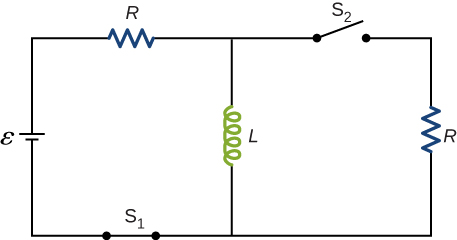a. 4.0 A; b. 2.4 A; c. on R : $V=12\phantom{\rule{0.2em}{0ex}}\text{V}$ ; on L : $V=7.9\phantom{\rule{0.2em}{0ex}}\text{V}$

The current in the RL circuit shown here increases to $40\text{%}$ of its steady-state value in 2.0 s. What is the time constant of the circuit?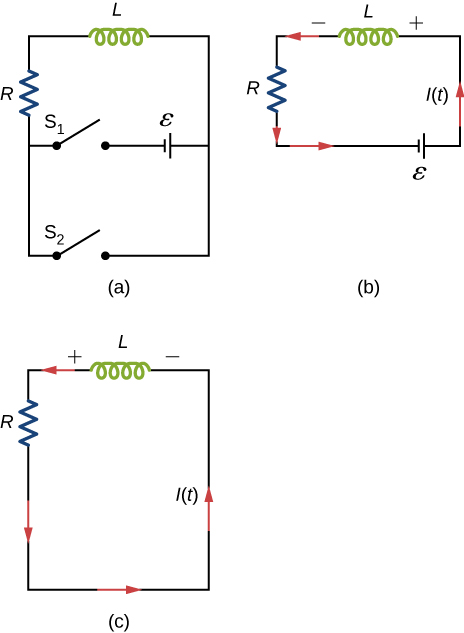How long after switch ${\text{S}}_{1}$ is thrown does it take the current in the circuit shown to reach half its maximum value? Express your answer in terms of the time constant of the circuit.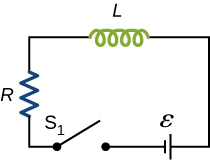$0.69\tau$

Examine the circuit shown below in part (a). Determine dI/dt at the instant after the switch is thrown in the circuit of (a), thereby producing the circuit of (b). Show that if I were to continue to increase at this initial rate, it would reach its maximum $\epsilon \text{/}R$ in one time constant.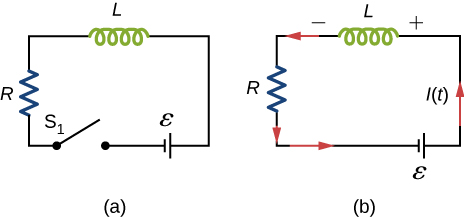The current in the RL circuit shown below reaches half its maximum value in 1.75 ms after the switch ${\text{S}}_{1}$ is thrown. Determine (a) the time constant of the circuit and (b) the resistance of the circuit if $L=250\phantom{\rule{0.2em}{0ex}}\text{mH}$ .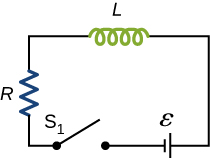a. 2.52 ms; b. $99.2\phantom{\rule{0.2em}{0ex}}\text{Ω}$

Consider the circuit shown below. Find ${I}_{1},{I}_{2},\phantom{\rule{0.2em}{0ex}}\text{and}\phantom{\rule{0.2em}{0ex}}{I}_{3}$ when (a) the switch S is first closed, (b) after the currents have reached steady-state values, and (c) at the instant the switch is reopened (after being closed for a long time).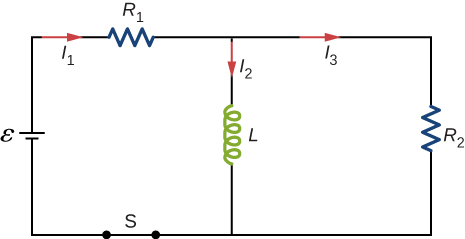For the circuit shown below, $\epsilon =50\phantom{\rule{0.2em}{0ex}}\text{V}$ , ${R}_{1}=10\phantom{\rule{0.2em}{0ex}}\text{Ω,}$ and $L=2.0\phantom{\rule{0.2em}{0ex}}\text{mH}$ . Find the values of ${I}_{1}\phantom{\rule{0.2em}{0ex}}\text{and}\phantom{\rule{0.2em}{0ex}}{I}_{2}$ (a) immediately after switch S is closed, (b) a long time after S is closed, (c) immediately after S is reopened, and (d) a long time after S is reopened.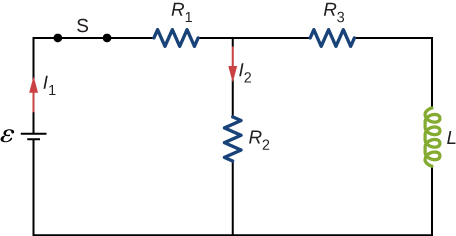a. ${I}_{1}={I}_{2}=1.7\phantom{\rule{0.2em}{0ex}}A$ ; b. ${I}_{1}=2.73\phantom{\rule{0.2em}{0ex}}A,{I}_{2}=1.36\phantom{\rule{0.2em}{0ex}}A$ ; c. ${I}_{1}=0,{I}_{2}=0.54\phantom{\rule{0.2em}{0ex}}A$ ; d. ${I}_{1}={I}_{2}=0$

For the circuit shown below, find the current through the inductor $2.0\phantom{\rule{0.2em}{0ex}}×\phantom{\rule{0.2em}{0ex}}{10}^{-5}\phantom{\rule{0.2em}{0ex}}\text{s}$ after the switch is reopened.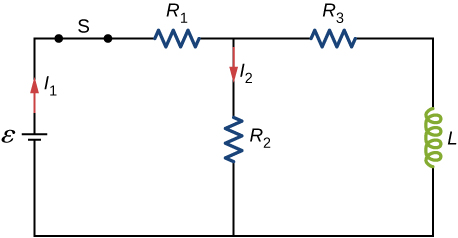Show that for the circuit shown below, the initial energy stored in the inductor, $L{I}^{2}\left(0\right)\text{/}2$ , is equal to the total energy eventually dissipated in the resistor, ${\int }_{0}^{\infty }{I}^{2}\left(t\right)Rdt$ .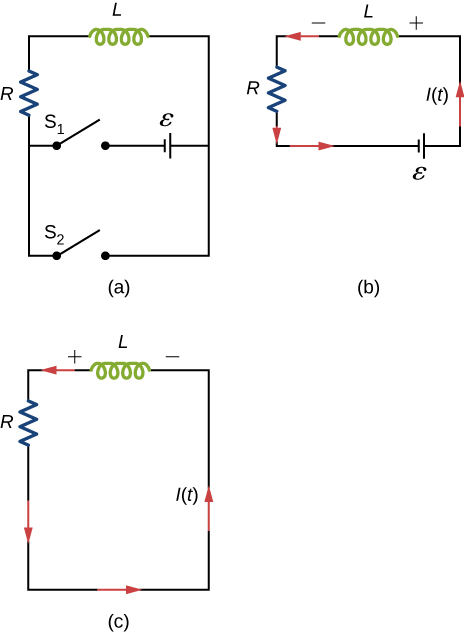proof

what is unit
is electric field directly proportional to the squared of a distance
The bullet 2.00cm long is fired at 420/s and passes straight through a 10.0 cm thick board existing at 280 m/s.What is the average acceleration of the bullet through the board?
FAUSTINA
an unstretched spring is 12cm long .A load of 5N stretched it to 15cm .how long will it be under a load of 15N?
Morapeli
hi
Africa
hi
Benjamin
Benjamin how are u are u a freshman in the university
Africa
like 100 level
Africa
yes sir
Benjamin
l need a physics tutor
Benjamin
I think the best tutor is God and organic tutor in YouTube that guy is good
Africa
me too 100level
Africa
wow nice
Benjamin
from Nigeria and u
Africa
I am from Nigeria and u wow nice that something I use to always say
Africa
am from Ghana
Benjamin
ok
Africa
studying what
Africa
Compare the electric flux through the surface of a cube of side length a that has a charge q at its center to the flux through a spherical surface of radius a with a charge q at its center.
please I want to know how to solve increase in length
Ujah
Why a charged capacitor has potential difference but not emf
what is the dimension symbol of temperature?
what is the dimension symbol of temperature?
Keren
what's the meaning of enthalpy in terms of latent heat, internal energy, phase change
how to convert Kelvin to centigrade
what is the s, p, d, f in this table
Sangeetha
s, p, d, f in this table
Sangeetha
what kind of table this
Vengata
what are waves
In physics, mathematics, and related fields, a wave is a propagating dynamic disturbance (change from equilibrium) of one or more quantities
Discuss how would orient a planar surface of area A in a uniform electric field of magnitude E0 to obtain (a) the maximum flux and (b) the minimum flux through the area.
I'm just doing the first 3 with this message. but thankyou for the time your obviously intending to support us with. viva la accumulation
Marcel
Find the net capacitance of the combination of series and parallel capacitors shown belo
what is ohm?
calculate ideal gas pressure of 0.300mol,v=2L T=40°c
what is principle of superposition
what are questions that are likely to come out during exam

#### Get Jobilize Job Search Mobile App in your pocket Now!By OpenStaxBy Edward BitonBy John GabrieliBy John GabrieliBy Anh DaoBy Brooke DelaneyBy OpenStaxBy OpenStaxBy Janet ForresterBy Jazzycazz Jackson